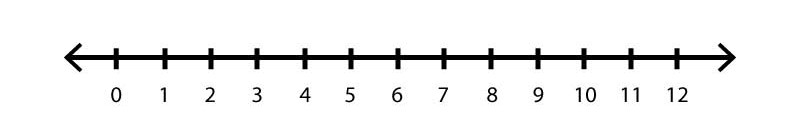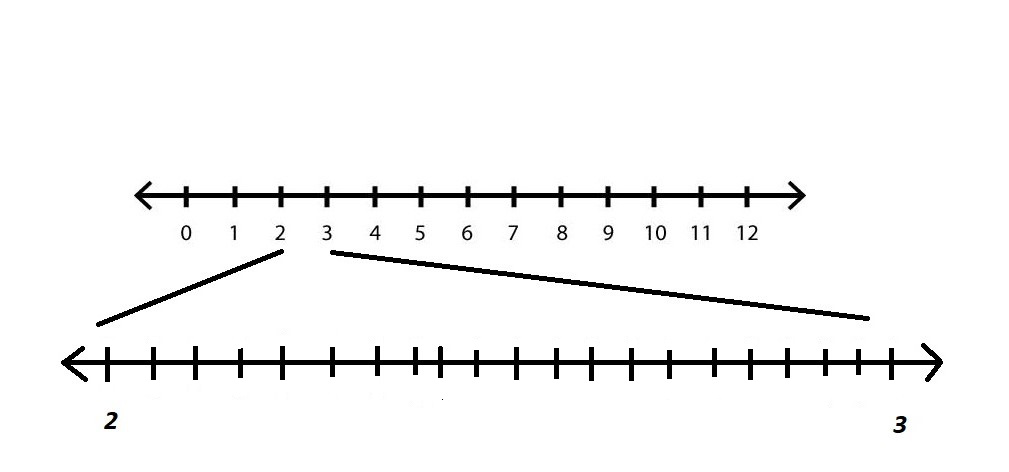# How Big is Infinity?

• Last Updated : 05 Aug, 2021

The simpler part about the word “Infinity” is that it does not have an end and since, there is no end defined for the word, the end is not required to be defined. The word Infinity is represented by the symbol ∞ which does not belong to any Integer, real number, fraction, etc. When “infinity” comes in picture, what number pops up in the head?

• Billion? – 10^9
• Googol? – 10^100
• Googolplex? –  10^Googol

But is this infinity? NO.

### Number Line

Lets understand the Number Line System first. It is a line on which numbers are placed at equidistant from each other. As a straight liner never ends, the number line too never ends. One can always think of the biggest number one could imagine, but adding 1 to it will make the number bigger. Thus it goes on and on.Number Line

Lets say, there are 12 cakes. It can be referred as having a dozen of cakes. Dozen is not a number but a size of a group or a set of cakes in this example. Dozen is a Cardinal number. In mathematics, Cardinal Number is the measure of a number of elements in the set.

In the same way, Infinity is a cardinal number representing the size of the all the numbers on the number line.

Few examples of Infinity:

• 1/3 = 0.33333…..
• ⨅ (pie) = 3.1415926….
• Infinity + Infinity = Infinity    so on and so forth.

### How large is Infinity?

A very intriguing question that rises with the word Infinity is How large is Infinity. Imagine there are infinite number of pens and are distributed to infinite number of people one by one in a queue. The cycle of giving pen to the people will never end even if people grow old and time pass, it will still continue. So far, the discussion concerned about the smallest type of Infinity (countable infinity), like the set of Natural numbers. If there is a set of infinite cakes and each cake can still be assigned to a natural number and people would never run out of natural numbers. Thus, a set of Natural numbers is a countably infinite set.

But one can have infinitely bigger set that contains all the numbers between two natural numbers, and this will be a bigger set than a set of natural numbers. Here, there are a group of numbers in between 2 and 3 which will be in decimals and the will never end (tends to infinity). Thus, infinity consists of infinite infinities.Set of numbers between 2 and 3

There can always have another bigger set that is not in one-to-one correspondence to the original set by just finding the collection of all the subsets of the initial set. This will be an infinite tower of infinity which leads to something called Absolute Infinity. One can only have a sense of what infinity is and its way more bigger than one could ever imagine.

In simple words, what’s beyond infinity?… is infinity again and so on.

Attention reader! All those who say programming isn’t for kids, just haven’t met the right mentors yet. Join the  Demo Class for First Step to Coding Coursespecifically designed for students of class 8 to 12.

The students will get to learn more about the world of programming in these free classes which will definitely help them in making a wise career choice in the future.

My Personal Notes arrow_drop_up